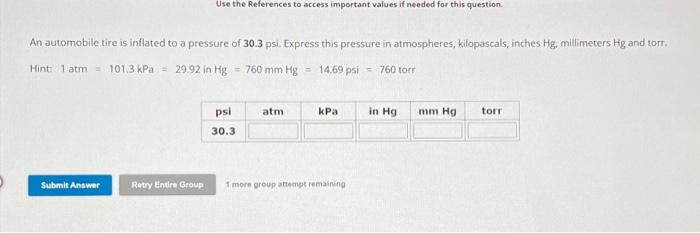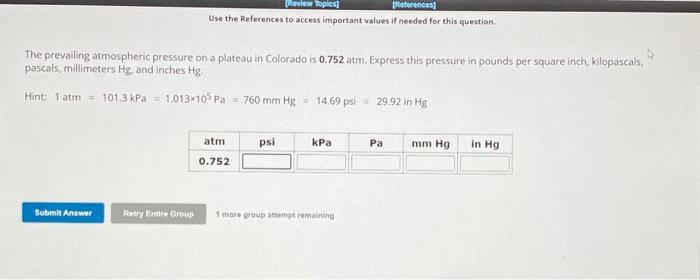Home / Expert Answers / Chemistry / an-automobile-tire-is-inflated-to-a-pressure-of-30-3-psi-express-this-pressure-in-atmospheres-kil-pa942

# (Solved): An automobile tire is inflated to a pressure of 30.3 psi. Express this pressure in atmospheres, kil ...An automobile tire is inflated to a pressure of 30.3 psi. Express this pressure in atmospheres, kilopascals, inches Hg, millimeters and torr. Hint torr Use the References to access important values if needed for this quention. The prevailing atmospheric pressure on a plateau in Colorado is 0.752 atm. Express this pressure in pounds per square inch. kilopascals, pascals, millimeters and inches . Hint:

We have an Answer from Expert

solution
given data
{A}
pressure of tire = 30.3 psi
hint : 1 atm = 101.3 kPa = 29.92 in Hg = 760 mmHg = 14.69 psi = 760 torr

now we calculate the value of each as:
{1}
1 atm = 14.69 psi
1 psi =    atm
30.3 psi =   30.3 atm
= 2.063 atm
{2}
1 atm = 101.3 kPa
2.063 atm = 2.063   101.3 kPa
We have an Answer from Expert